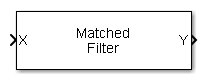# Matched Filter

Matched filter

•Libraries:
Phased Array System Toolbox / Detection

## Description

The Matched Filter block implements matched filtering of an input signal. Matched filtering is an FIR filtering operation with the coefficients equal to the time reversed samples of the transmitted signal. The filter can improve SNR before detection.

## Ports

### Input

expand all

Input signal, specified as an M-by-N complex valued matrix. Matched filtering is applied along the first dimension. The input `X` and the output `Y` have the same dimensions. The initial transient is removed from the filtered result.

The size of the first dimension of the input matrix can vary to simulate a changing signal length. A size change can occur, for example, in the case of a pulse waveform with variable pulse repetition frequency.

Data Types: `double`

Matched filter coefficients, specified as an M-length complex column vector.

#### Dependencies

To enable this port, set the Source of coefficients pull-down menu ```Input port```.

Data Types: `double`

### Output

expand all

Matched filter output, returned as an M-by-N complex valued matrix. The dimensions of `Y` are the same as the dimensions of `X`.

Data Types: `double`

Matched-filter gain, returned as an N real-valued matrix. Gain is computed for each column of `X`. Units are in dB.

#### Dependencies

To enable this port, select the Enable SNR gain output check box.

Data Types: `double`

## Parameters

expand all

Specify whether the matched filter coefficients come from Coefficients or from an input port.

 `Property` Matched filter coefficients are specified by Coefficients . `Input port` Matched filter coefficients are specified via the input port `Coeff` .

Specify the matched filter coefficients as a column vector. This parameter appears when you set Source of coefficients to `Property`.

Specify the window used for spectrum weighting using one of

 `None` `Hamming` `Chebyshev` `Hann` `Kaiser` `Taylor`

Spectrum weighting is often used with linear FM waveforms to reduce sidelobe levels in the time domain. The block computes the window length internally to match the FFT length.

Specify the spectrum region, in hertz, on which the spectrum window is applied as a 1-by-2 vector in the form of `[StartFrequency,EndFrequency]`.

Note that both `StartFrequency` and `EndFrequency` are measured in baseband. That is, they are within `[-Fs/2,Fs/2]`, where `Fs` is the sample rate specified in any of the waveform library blocks. The parameter `StartFrequency` must be less than `EndFrequency`.

#### Dependencies

This parameter appears when you set the Spectrum window parameter to any value other than `None`.

Select this parameter to inherit the sample rate from upstream blocks. Otherwise, specify the sample rate using the Sample rate (Hz) parameter.

Data Types: `Boolean`

Sample rate of the output waveform, specified as a positive scalar. The ratio of Sample rate (Hz) to each element in the Pulse repetition frequency (Hz) vector must be an integer. This restriction is equivalent to requiring that the pulse repetition interval is an integral multiple of the sample interval.

#### Programmatic Use

 Block Parameter:`SampleRate` Type:double Values:positive scalar Default:`1e6`

Specify the sidelobe attenuation level, in dB, of a Chebyshev or Taylor window as a positive scalar.

#### Dependencies

This parameter appears when you set Spectrum window to `Chebyshev` or `Taylor`.

Specify the parameter that affects the Kaiser window sidelobe attenuation as a nonnegative scalar. Please refer to the function `kaiser` for more details.

#### Dependencies

This parameter appears when you set the Spectrum window parameter to `Kaiser`.

Specify the number of nearly-constant-level sidelobes adjacent to the mainlobe in a Taylor window as a positive integer.

#### Dependencies

This parameter appears when you set the Spectrum window parameter to `Taylor`.

Select this check this box to obtain the matched filter SNR gain via the output port `G`. The output port appears only when this box is selected.

Block simulation, specified as `Interpreted Execution` or `Code Generation`. If you want your block to use the MATLAB® interpreter, choose `Interpreted Execution`. If you want your block to run as compiled code, choose ```Code Generation```. Compiled code requires time to compile but usually runs faster.

Interpreted execution is useful when you are developing and tuning a model. The block runs the underlying System object™ in MATLAB. You can change and execute your model quickly. When you are satisfied with your results, you can then run the block using ```Code Generation```. Long simulations run faster with generated code than in interpreted execution. You can run repeated executions without recompiling, but if you change any block parameters, then the block automatically recompiles before execution.

This table shows how the Simulate using parameter affects the overall simulation behavior.

When the Simulink® model is in `Accelerator` mode, the block mode specified using Simulate using overrides the simulation mode.

Acceleration Modes

 Block Simulation Simulation Behavior `Normal` `Accelerator` `Rapid Accelerator` `Interpreted Execution` The block executes using the MATLAB interpreter. The block executes using the MATLAB interpreter. Creates a standalone executable from the model. `Code Generation` The block is compiled. All blocks in the model are compiled.

 Block Parameter:`SimulateUsing` Type:enum Values:```Interpreted Execution```, `Code Generation` Default:```Interpreted Execution```